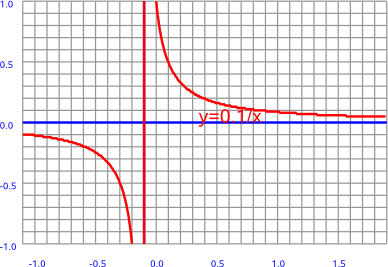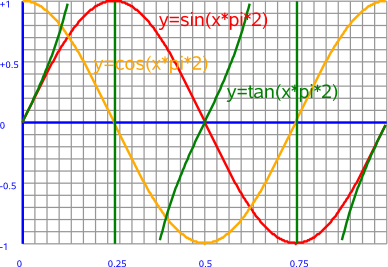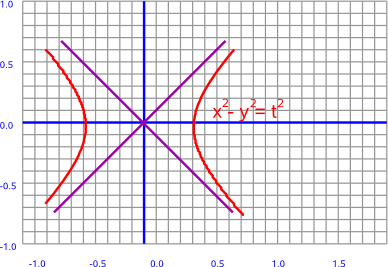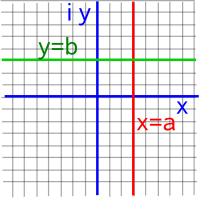# Maths - Functions

These pages deal with continuous functions such as:

## Scalar Functions

Here we are looking at functions that map one scalar value to another scalar value. In order to see the function graphically we can show all possible input values as the horizontal axis and the output values as the vertical axis. The translation between the two can then be drawn as a (possibly) curved line.

terminology:

• scalar
• domain
• co-domain

A function f maps elements from domain A to codomain B.This is denoted as follows,

f: A → B

### Singularities

A singularity is a single point in a function where it does not behave in a continuous way, for example, a step function. Or where the function goes to infinity. There are also more subtle things like a point where the function does not have an inverse and if there are multiple inputs, there may be a point where the inputs are couples and not independent.

### Calculating the Value of a Function

If we want to calculate the value of a function for a specific input value we can often use an infinite series. This is often used in devices like calculators and computers to evaluate the value of a function. Although such devices can't calculate an infinite number of terms and therefore don't give the exact value they can get any level of accuracy required by taking enough terms.

 f(x) f(0) + x f'(0) + x2/2! f''(0)+ x3/3! f'''(0) ... Maclaurins series f(x + h) f(x) + h f'(x) + h2/2! f''(x)+ h3/3! f'''(x) ... Taylors series

### Inverse FunctionWe can see that, for this function, every value of x produces a value of y.

However there is one value on the graph, at x=0, where something strange happens. The value of y jumps from -∞ to +∞ at a single point. This is known as a singularity.

### Log and Exponential Functionsln(1+x) x - x2/2! + x3/3! ... +(-1)r+1xr/(r)! -1 < x <= 1 exp(x) 1 + x1/1! + x2/2! + x3/3! ... + xr/(r)! all values of x exp(-x) 1 - x1/1! + x2/2! - x3/3! ... all values of x e 1 + 1/1! + 2/2! + 3/3! =2.718281828

### Trigometric FunctionsTrig functions are

 sin(x) x - x3/3! + x5/5! ... +(-1)rx2r+1/(2r+1)! all values of x cos(x) 1 - x2/2! + x4/4! ... +(-1)rx2r/(2r)! all values of x

## Circular Function

If we have an equation like:

x2 + y2 = r2

then the graph is a circle.## Hyperbolic Function

If we have an equation like:

x2 - y2 = t2

then the graph is a hyperbolic function.This is the 'signature' of Minkowski space, the simplest example would be double numbers which are like a world with one dimension of space and one dimension of time.

 sinh(x) x + x3/3! + x5/5! ... +x2r+1/(2r+1)! all values of x cosh(x) 1 + x2/2! + x4/4! ... +x2r/(2r)! all values of x

## Functions in Higher Dimensions

With a higher dimensional quantity like a complex number we need to map every point in a plane to a point in another plane. This is much more difficult to show graphically, one way to do this is to show how horizontal (green) and vertical (red) lines are transformed as shown here for the square function:

z plane   w plane-->

w=z²algebra dimensions
Complex Number Functions 2
Double Number Functions 2
Dual Number Functions 2
Quaternion Functions 4
Matrix Functions
Clifford Algebra Functions

 metadata block see also: Correspondence about this page Book Shop - Further reading. Where I can, I have put links to Amazon for books that are relevant to the subject, click on the appropriate country flag to get more details of the book or to buy it from them.The MathML Handbook - for people interested in working with mathematics on the web. Other Math Books Specific to this page here:

This site may have errors. Don't use for critical systems.